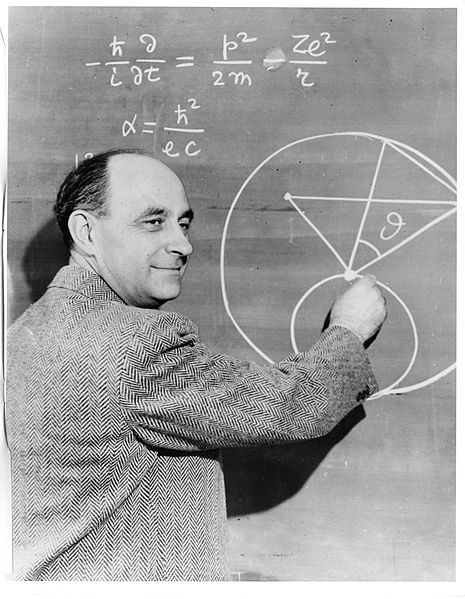# I Find Blackboards Cool

Probability Level 4I have an infinite number of $1's$ written on a blackboard.

Jake chooses $2$ of the integers $p$ and $q$ and replaces them with $\dfrac{p+q}{4}={ k }_{ 1 }$. (he removes $p$ and $q$ and then writes $\dfrac{p+q}{4}$)

Now he repeats the process with the number ${ k }_{ 1 }$ and another integer to achieve ${ k }_{ 2 }$, and repeats again with the number ${ k }_{ 2 }$ and another integer to achieve ${ k }_{ 3 }$

${ k }_{ 2 }=\frac { 1+{ k }_{ 1 } }{ 4 } ,\quad { k }_{ 3 }=\frac { 1+{ k }_{ 2 } }{ 4 }$

Since he is an immortal, he does this again and again until he is left with only a single number...

Given that this number can be expressed as $\frac{a}{b}$, find $a+b$

Try my Other Problems

×

Problem Loading...

Note Loading...

Set Loading...